Log Service allows you to use different types of date and time functions to process log data. The functions include time functions, date functions, date and time extraction functions, time interval functions, and time series padding functions. You can use the functions to convert the format of date and time in logs. You can also use the functions to group and aggregate logs. This topic describes the syntax of date and time functions. This topic also provides examples on how to use date and time functions.

Notice
• The timestamp of a log in Log Service is accurate to the second. Therefore, you can specify the time format only to the second. For more information, see Formats.
• You need to specify the time format only for the time in a time string. You do not need to specify the time format for other parameters such as the time zone. For more information, see Formats.
• Each log in Log Service contains the reserved __time__ field. The value of the field is a UNIX timestamp. Example: 1592374067, which indicates 2020-06-17 14:07:47.
• If you want to use strings in analytic statements, you must enclose the strings in single quotation marks (''). Strings that are not enclosed or are enclosed in double quotation marks ("") indicate field names or column names. For example, 'status' indicates the status string, and status or "status" indicates the status log field.
Type Function Syntax Description
Date and time functions current_date function current_date Returns the current date.
current_time function current_time Returns the current time and time zone.
current_timestamp function current_timestamp Returns the current date, time, and time zone.
current_timezone function current_timezone() Returns the current time zone.
date function date(x) Returns the date part of a datetime expression.
date_format function date_format(x,format) Converts a datetime expression that can return a timestamp value to a datetime expression in a specified format.
date_parse function date_parse(x,format) Converts a datetime string to a datetime expression that can return a timestamp value and is in a specified format.
from_iso8601_date function from_iso8601_date(x) Converts a date expression in the ISO 8601 format to a date expression that can return a date value.
from_iso8601_timestamp function from_iso8601_timestamp(x) Converts a datetime expression in the ISO 8601 format to a datetime expression that can return a timestamp value.
from_unixtime function from_unixtime(x) Converts a UNIX timestamp to a datetime expression that can return a timestamp value and does not contain a time zone.
from_unixtime(x,time zone) Converts a UNIX timestamp to a datetime expression that can return a timestamp value and contains a time zone.
from_unixtime(x,hours,minutes) Converts a UNIX timestamp to a datetime expression that can return a timestamp value and contains a time zone. In the datetime expression, the values for hours and minutes indicate the offset of the time zone.
localtime function localtime Returns the local time.
localtimestamp function localtimestamp Returns the local date and time.
now function now() Returns the current date and time.

This function is equivalent to the current_timestamp function.

to_iso8601 function to_iso8601(x) Converts a datetime expression that can return a date or timestamp value to a datetime expression in the ISO 8601 format.
to_unixtime function to_unixtime(x) Converts a datetime expression that can return a timestamp value to a UNIX timestamp.
Date and time extraction functions day function day(x) Returns the day of the month from a datetime expression.

This function is equivalent to the day_of_month function.

day_of_month function day_of_month(x) Returns the day of the month from a datetime expression.

This function is equivalent to the day function.

day_of_week function day_of_week(x) Returns the day of the week from a datetime expression.

This function is equivalent to the dow function.

day_of_year function day_of_year(x) Returns the day of the year from a datetime expression.

This function is equivalent to the doy function.

dow function dow(x) Returns the day of the week from a datetime expression.

This function is equivalent to the day_of_week function.

doy function doy(x) Returns the day of the year from a datetime expression.

This function is equivalent to the day_of_year function.

extract function extract(field from x) Returns the specified field from a datetime expression. The field can be a date or time.
hour function hour(x) Returns the hour of the day from a datetime expression. The 24-hour clock is used.
minute function minute(x) Returns the minute of the hour from a datetime expression.
month function month(x) Returns the month of the year from a datetime expression.
quarter function quarter(x) Returns the quarter of the year on which a specified date falls.
second function second(x) Returns the second of the minute from a datetime expression.
timezone_hour function timezone_hour(x) Returns the offset of the time zone in hours.
timezone_minute function timezone_minute(x) Returns the offset of the time zone in minutes.
week function week(x) Returns the week of the year on which a specified date falls.

This function is equivalent to the week_of_year function.

week_of_year function week_of_year(x) Returns the week of the year on which a specified date falls.

This function is equivalent to the week function.

year function year(x) Returns the year of a specified date.
year_of_week function year_of_week(x) Returns the year on which a specified date falls in the ISO week date system.

This function is equivalent to the yow function.

yow function yow(x) Returns the year on which a specified date falls in the ISO week date system.

This function is equivalent to the year_of_week function.

Time interval functions date_trunc function date_trunc(unit,x) Truncates a datetime expression based on the time unit that you specify. The expression can be truncated based on the millisecond, second, minute, hour, day, month, or year.
date_add function date_add(unit, N,x) Adds N to the value of the x field based on the unit that you specify.
date_diff function date_diff(unit, x, y) Returns the difference between two time expressions. For example, you can calculate the difference between x and y based on the time unit that you specify.
Time series padding function time_series function time_series(x, window, format, padding_data) Adds a value to the field that has no value returned in the specified time window.

## current_date function

The current_date function returns the current date. The return value is in the YYYY-MM-DD format.

### Syntax

``current_date``

The date type.

### Examples

Query the logs of the previous day.

• Query statement
``````* |
SELECT
*
FROM  log
WHERE
__time__ < to_unixtime(current_date)
AND __time__ > to_unixtime(date_add('day', -1, current_date))``````
• Query and analysis results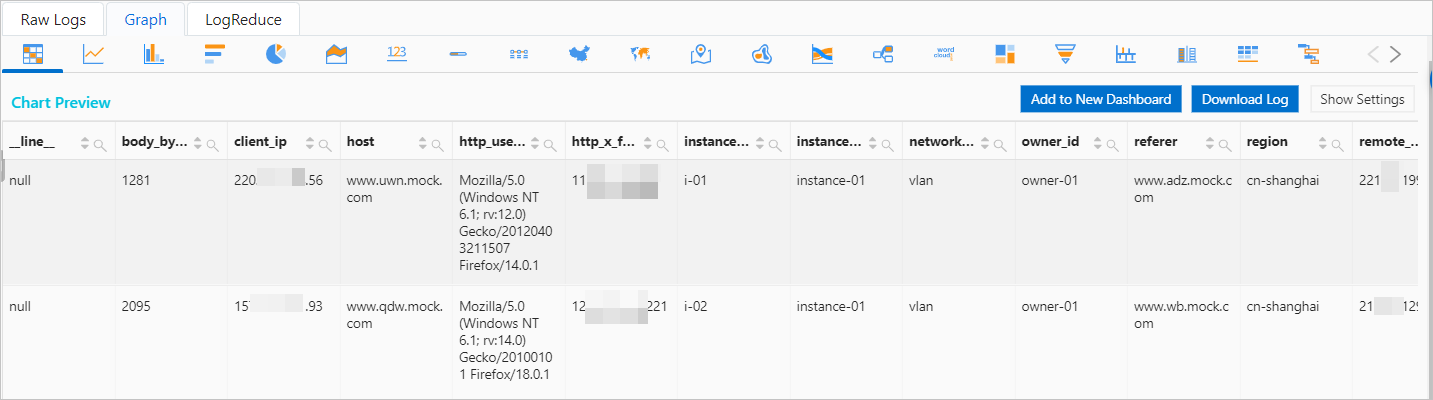## current_time function

The current_time function returns the current time and time zone. The return value is in the HH:MM:SS.Ms Time_zone format.

### Syntax

``current_time``

The time type.

### Examples

Query the current time and time zone.

• Query statement
``* | select current_time``
• Query and analysis results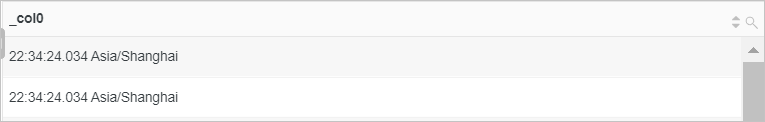## current_timestamp function

The current_timestamp function returns the current date, time, and time zone. The return value is in the YYYY-MM-DD HH:MM:SS.Ms Time_zone format.

### Syntax

``current_timestamp``

### Return value type

The timestamp type.

### Examples

Query the logs of the previous day.

• Query statement
``````* |
SELECT
*
FROM  log
WHERE
__time__ < to_unixtime(current_timestamp)
AND __time__ > to_unixtime(date_add('day', -1, current_timestamp))``````
• Query and analysis results## current_timezone function

The current_timezone function returns the current time zone.

### Syntax

``current_timezone()``

### Return value type

The varchar type.

### Examples

Query the current time zone.

• Query statement
``* | select current_timezone()``
• Query and analysis results## date function

The date function returns the date part of a datetime expression. This function is equivalent to the `cast(x as date)` function. For more information, see Data type conversion functions.

### Syntax

``date(x)``

### Parameters

Parameter Description
x The value of this parameter is of the date or timestamp type.

The date type.

### Examples

Use the current_timestamp function to obtain the current date and time. Then, use the date function to obtain the date part of the current date and time.

• Query statement
``* | SELECT current_timestamp, date(current_timestamp)``
• Query and analysis results## date_format function

The date_format function converts a datetime expression that can return a timestamp value to a datetime expression in a specified format.

### Syntax

``date_format(x,format)``

### Parameters

Parameter Description
x The value of this parameter is a datetime expression that can return a timestamp value.
format The format of the datetime expression to which you want to convert a datetime expression that can return a timestamp value. For more information, see Formats.

### Return value type

The varchar type.

### Examples

Query the status of NGINX requests, calculate the number of NGINX requests, and then display the query and analysis results in chronological order. To do this, use the date_trunc function to truncate the log time by minute and use the date_format function to convert the time to the `%H:%i` format. Then, calculate the number of requests for each status code per minute and display the query and analysis results in a flow chart.

• Query statement
``````* |
SELECT
date_format(date_trunc('minute', __time__), '%H:%i') AS time,
COUNT(1) AS count,
status
GROUP BY
time,
status
ORDER BY
time``````
• Query and analysis results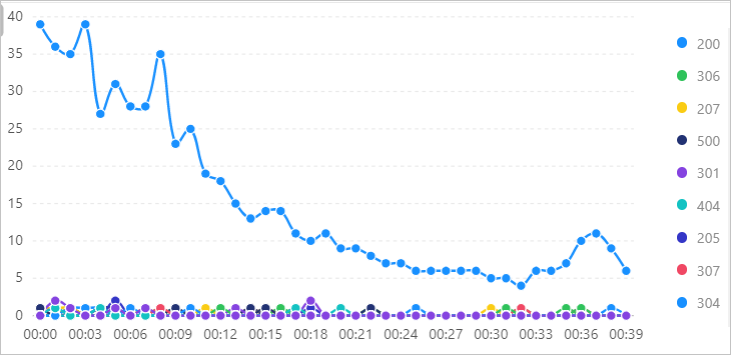## date_parse function

The date_parse function converts a datetime string to a datetime expression that can return a timestamp value and is in a specified format.

### Syntax

``date_parse(x,format)``

### Parameters

Parameter Description
x The value of this parameter is a datetime string.
format The format of the datetime expression that can return a timestamp value to which you want to convert the datetime string. For more information, see Formats.

### Return value type

The timestamp type.

### Examples

Convert the values of the StartTime and EndTime fields to datetime expressions that can return a timestamp value and calculate the difference between the two datetime expressions.

• Query statement
``````*|
SELECT
date_parse(StartTime, '%Y-%m-%d %H:%i') AS "StartTime",
date_parse(EndTime, '%Y-%m-%d %H:%i') AS "EndTime",
date_diff('hour', StartTime, EndTime) AS "Time difference (hour)"``````
• Query and analysis results## from_iso8601_date function

The from_iso8601_date function converts a date expression in the ISO 8601 format to a date expression that can return a date value. The return value is in the YYYY-MM-DD format.

### Syntax

``from_iso8601_date(x)``

### Parameters

Parameter Description
x The value of this parameter is a date expression in the ISO 8601 format.

The date type.

### Examples

Convert the value of the time field to a date expression that can return a date value

• Sample field
``time:2020-05-03``
• Query statement
``* | select from_iso8601_date(time)``
• Query and analysis results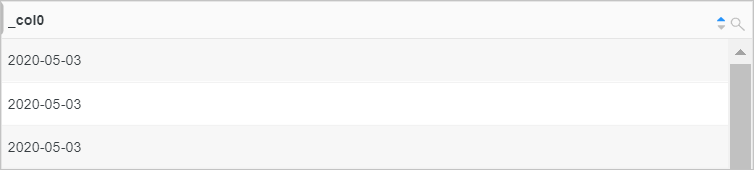## from_iso8601_timestamp function

The from_iso8601_timestamp function converts a datetime expression in the ISO 8601 format to a datetime expression that can return a timestamp value. The return value is in the YYYY-MM-DD HH:MM:SS.Ms Time_zone format.

### Syntax

``from_iso8601_timestamp(x)``

### Parameters

Parameter Description
x The value of this parameter is a datetime expression in the ISO 8601 format.

### Return value type

The timestamp type.

### Examples

Convert the value of the time field to a datetime expression that can return a timestamp value.

• Sample field
``time:2020-05-03T17:30:08``
• Query statement
``* | select from_iso8601_timestamp(time)``
• Query and analysis results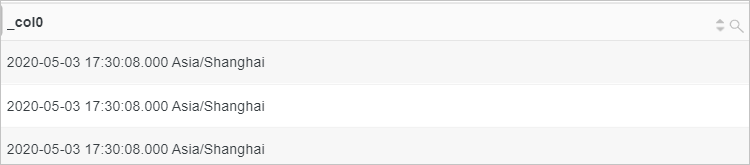## from_unixtime function

The from_unixtime function converts a UNIX timestamp to a datetime expression that can return a timestamp value. The return value is in the YYYY-MM-DD HH:MM:SS.Ms or YYYY-MM-DD HH:MM:SS.Ms Time_zone format.

### Syntax

• The following function converts a UNIX timestamp to a datetime expression that can return a timestamp value and does not contain a timezone.
``from_unixtime(x)``
• The following function converts a UNIX timestamp to a datetime expression that can return a timestamp value and contains a timezone.
``from_unixtime(x,time zone)``
• The following function converts a UNIX timestamp to a datetime expression that can return a timestamp value and contains a timezone. In the datetime expression, the values for hours and minutes indicate the offset of the time zone.
``from_unixtime(x,hours,minutes)``

### Parameters

Parameter Description
x The value of this parameter is a UNIX timestamp.
time zone The time zone. Example: Asia/shanghai.
hours The offset of the time zone in hours. Examples: +07 and -09.
minutes The offset of the time zone in minutes. Examples: +30 and -45.

### Return value type

The timestamp type.

### Examples

Convert the value of the time field to a datetime expression that can return a timestamp value and contains a timezone.

• Sample field
``time:1626774758``
• Query statement
``* | select from_unixtime(time,'Asia/shanghai')``
• Query and analysis results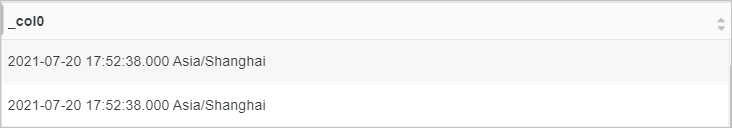## localtime function

The localtime function returns the local time. The return value is in the HH:MM:SS.Ms format.

### Syntax

``localtime``

The time type.

### Examples

Query the local time.

• Query statement
``* | select localtime``
• Query and analysis results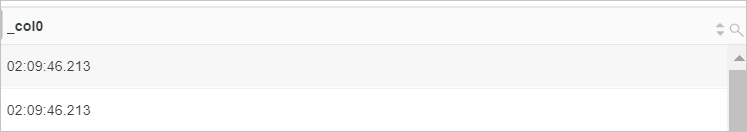## localtimestamp function

The localtimestamp function returns the local date and time. The return value is in the YYYY-MM-DD HH:MM:SS.Ms Time_zone format.

### Syntax

``localtimestamp``

### Return value type

The timestamp type.

### Examples

Query the logs of the previous day.

• Query statement
``````* |
SELECT
*
FROM  log
WHERE
__time__ < to_unixtime(localtimestamp)
AND __time__ > to_unixtime(date_add('day', -1, localtimestamp))``````
• Query and analysis results## now function

The now function returns the current date and time. The return value is in the YYYY-MM-DD HH:MM:SS.Ms Time_zone format. This function is equivalent to the current_timestamp function.

### Syntax

``now()``

### Return value type

The timestamp type.

### Examples

Query the logs of the previous day.

• Query statement
``````* |
SELECT
*
FROM  log
WHERE
__time__ < to_unixtime(now())
AND __time__ > to_unixtime(date_add('day', -1, now()))``````
• Query and analysis results## to_iso8601 function

The to_iso8601 function converts a datetime expression that can return a date or timestamp value to a datetime expression in the ISO 8601 format.

### Syntax

``to_iso8601(x)``

### Parameters

Parameter Description
x The value of this parameter is of the date or timestamp type.

### Return value type

The varchar type.

### Examples

Use the current_timestamp function to obtain the current date and time. Then, use the to_iso8601 function to convert the current date and time to the ISO 8601 format.

• Query statement
``* | select to_iso8601(current_timestamp) AS ISO8601``
• Query and analysis results## to_unixtime function

The to_unixtime function converts a datetime expression that can return a timestamp value to a UNIX timestamp.

### Syntax

``to_unixtime(x)``

### Parameters

Parameter Description
x The value of this parameter is a datetime expression that can return a timestamp value.

The double type.

### Examples

Query the logs of the previous day.

• Query statement
``````* |
SELECT
*
FROM  log
WHERE
__time__ < to_unixtime(now())
AND __time__ > to_unixtime(date_add('day', -1, now()))``````
• Query and analysis results## day function

The day function returns the day of the month from a datetime expression. This function is equivalent to the day_of_month function.

### Syntax

``day(x)``

### Parameters

Parameter Description
x The value of this parameter is of the timestamp or date type.

The bigint type.

### Examples

Use the current_date function to obtain the current date. Then, use the day function to obtain the day of the month based on the current date.

• Query statement
``* | SELECT current_date, day(current_date)``
• Query and analysis results## day_of_month function

The day_of_month function returns the day of the month from a datetime expression. This function is equivalent to the day function.

### Syntax

``day_of_month(x)``

### Parameters

Parameter Description
x The value of this parameter is of the timestamp or date type.

The bigint type.

### Examples

Use the current_date function to obtain the current date. Then, use the day_of_month function to obtain the day of the month based on the current date.

• Query statement
``* | SELECT current_date, day_of_month(current_date)``
• Query and analysis results## day_of_week function

The day_of_week function returns the day of the week from a datetime expression.

### Syntax

``day_of_week(x)``

### Parameters

Parameter Description
x The value of this parameter is of the timestamp or date type.

The bigint type.

### Examples

Use the current_date function to obtain the current date. Then, use the day_of_week function to obtain the day of the week based on the current date.

• Query statement
``* | SELECT current_date, day_of_week(current_date)``
• Query and analysis results## day_of_year function

The day_of_year function returns the day of the year from a datetime expression.

### Syntax

``day_of_year(x)``

### Parameters

Parameter Description
x The value of this parameter is of the timestamp or date type.

The bigint type.

### Examples

Use the current_date function to obtain the current date. Then, use the day_of_year function to obtain the day of the year based on the current date.

• Query statement
``* | SELECT current_date, day_of_year(current_date)``
• Query and analysis results## dow function

The dow function returns the day of the week from a datetime expression. This function is equivalent to the day_of_week function.

### Syntax

``dow(x)``

### Parameters

Parameter Description
x The value of this parameter is of the timestamp or date type.

The bigint type.

### Examples

Use the current_date function to obtain the current date. Then, use the dow function to obtain the day of the week based on the current date.

• Query statement
``* | SELECT current_date, dow(current_date)``
• Query and analysis results## doy function

The doy function returns the day of the year from a datetime expression. This function is equivalent to the day_of_year function.

### Syntax

``doy(x)``

### Parameters

Parameter Description
x The value of this parameter is of the timestamp or date type.

The bigint type.

### Examples

Use the current_date function to obtain the current date. Then, use the doy function to obtain the day of the year based on the current date.

• Query statement
``* | SELECT current_date, doy(current_date)``
• Query and analysis results## extract function

The extract function returns the specified field from a datetime expression. The field can be a date or a time.

### Syntax

``extract(field from x)``

### Parameters

Parameter Description
field Valid values: year, quarter, month, week, day, day_of_month, day_of_week, dow, day_of_year, doy, year_of_week, yow, hour, minute, second, timezone_hour, and timezone_minute.
x The value of this parameter is of the date, time, timestamp, or interval (actual varchar(9)) type.

The bigint type.

### Examples

Use the current_date function to obtain the current date. Then, use the extract function to obtain the year of the current date.

• Query statement
``* | SELECT extract(year from current_date)``
• Query and analysis results## hour function

The hour function returns the hour of the day from a datetime expression. The 24-hour clock is used.

### Syntax

``hour(x)``

### Parameters

Parameter Description
x The value of this parameter is of the timestamp type.

The bigint type.

### Examples

Use the current_timestamp function to obtain the current date and time. Then, use the hour function to obtain the hour of the day based on the current time.

• Query statement
``* | SELECT current_timestamp, hour(current_timestamp)``
• Query and analysis results## minute function

The minute function returns the minute of the hour from a datetime expression.

### Syntax

``minute(x)``

### Parameters

Parameter Description
x The value of this parameter is of the timestamp type.

The bigint type.

### Examples

Use the current_timestamp function to obtain the current date and time. Then, use the minute function to obtain the minute of the hour based on the current time.

• Query statement
``* | SELECT current_timestamp, minute(current_timestamp)``
• Query and analysis results## month function

The month function returns the month of the year from a datetime expression.

### Syntax

``month(x)``

### Parameters

Parameter Description
x The value of this parameter is of the date or timestamp type.

The bigint type.

### Examples

Use the current_timestamp function to obtain the current date and time. Then, use the month function to obtain the month of the year based on the current date.

• Query statement
``* | SELECT current_timestamp, month(current_timestamp)``
• Query and analysis results## quarter function

The quarter function returns the quarter of the year on which a specified date falls.

### Syntax

``quarter(x)``

### Parameters

Parameter Description
x The value of this parameter is of the date or timestamp type.

The bigint type.

### Examples

Use the current_timestamp function to obtain the current date and time. Then, use the quarter function to obtain the quarter of the year on which the current date falls.

• Query statement
``* | SELECT current_timestamp,quarter(current_timestamp)``
• Query and analysis results## second function

The second function returns the second of the minute from a datetime expression.

### Syntax

``second(x)``

### Parameters

Parameter Description
x The value of this parameter is of the timestamp type.

The bigint type.

### Examples

Use the current_timestamp function to obtain the current date and time. Then, use the second function to obtain the second of the minute based on the current time.

• Query statement
``* | SELECT current_timestamp,second(current_timestamp)``
• Query and analysis results## timezone_hour function

The timezone_hour function returns the offset of the time zone in hours.

### Syntax

``timezone_hour(x)``

### Parameters

Parameter Description
x The value of this parameter is of the timestamp type.

The bigint type.

### Examples

Use the current_timestamp function to obtain the current date and time. Then, use the timezone_hour function to obtain the offset of the time zone to which the current time belongs in hours.

• Query statement
``* | SELECT current_timestamp,timezone_hour(current_timestamp)``
• Query and analysis results## timezone_minute function

The timezone_minute function returns the offset of the time zone in minutes.

### Syntax

``timezone_minute(x)``

### Parameters

Parameter Description
x The value of this parameter is of the timestamp type.

The bigint type.

### Examples

Use the current_timestamp function to obtain the current date and time. Then, use the timezone_minute function to obtain the offset of the time zone to which the current time belongs in minutes.

• Query statement
``* | SELECT current_timestamp,timezone_minute(current_timestamp)``
• Query and analysis results## week function

The week function returns the week of the year on which a specified date falls. This function is equivalent to the week_of_year function.

### Syntax

``week(x)``

### Parameters

Parameter Description
x The value of this parameter is of the date or timestamp type.

The bigint type.

### Examples

Use the current_timestamp function to obtain the current date and time. Then, use the week function to obtain the week of the year on which the current date falls.

• Query statement
``* | SELECT current_timestamp,week(current_timestamp)``
• Query and analysis results## week_of_year function

The week_of_year function returns the week of the year on which a specified date falls. This function is equivalent to the week function.

### Syntax

``week_of_year(x)``

### Parameters

Parameter Description
x The value of this parameter is of the date or timestamp type.

The bigint type.

### Examples

Use the current_timestamp function to obtain the current date and time. Then, use the week_of_year function to obtain the week of the year on which the current date falls.

• Query statement
``* | SELECT current_timestamp,week_of_year(current_timestamp)``
• Query and analysis results## year function

The year function returns the year of a specified date.

### Syntax

``year(x)``

### Parameters

Parameter Description
x The value of this parameter is of the date or timestamp type.

The bigint type.

### Examples

Use the current_timestamp function to obtain the current date and time. Then, use the year function to obtain the year of the current date.

• Query statement
``* | SELECT current_timestamp,year(current_timestamp)``
• Query and analysis results## year_of_week function

The year_of_week function returns the year on which a specified date falls in the ISO week date system. This function is equivalent to the yow function.

### Syntax

``year_of_week(x)``

### Parameters

Parameter Description
x The value of this parameter is of the date or timestamp type.

The bigint type.

### Examples

Use the current_timestamp function to obtain the current date and time. Then, use the year_of_week function to obtain the year on which the current date falls in the ISO week date system.

• Query statement
``* | SELECT current_timestamp,year_of_week(current_timestamp)``
• Query and analysis results## yow function

The yow function returns the year on which a specified date falls in the ISO week date system. This function is equivalent to the year_of_week function.

### Syntax

``yow(x)``

### Parameters

Parameter Description
x The value of this parameter is of the date or timestamp type.

The bigint type.

### Examples

Use the current_timestamp function to obtain the current date and time. Then, use the yow function to obtain the year on which the current date falls in the ISO week date system.

• Query statement
``* | SELECT current_timestamp,yow(current_timestamp)``
• Query and analysis results## date_trunc function

The date_trunc function truncates a datetime expression based on the time unit that you specify. The expression can be truncated based on the millisecond, second, minute, hour, day, month, or year. This function is often used in scenarios that require statistical analysis by time.

### Syntax

``date_trunc(unit,x)``

### Parameters

Parameter Description
unit The unit of time. Valid values: millisecond, second, minute, hour, day, week, month, quarter, and year. For more information, see Units.
x The value of this parameter is a datetime expression.
Note The date_trunc function allows you to measure statistics only based on a fixed interval. The interval is determined by the time unit that you specify in the function. The time unit includes the minute or hour. If you want to measure statistics based on a custom interval, we recommend that you perform a mathematical modulo operation to group data. For example, a mathematical modulo operation is performed to group data at 5-minute intervals.
``* | SELECT count(1) AS pv,  __time__ - __time__ %300 AS time GROUP BY time LIMIT 100``

### Return value type

Same as the data type of the parameter value.

### Examples

Calculate an average request duration by minute. Then, group and sort the durations in chronological order.

• Query statement
``````* |
SELECT
date_trunc('minute', __time__) AS time,
truncate (avg(request_time)) AS avg_time,
current_date AS date
GROUP BY
time
ORDER BY
time DESC
LIMIT
100``````
• Query and analysis results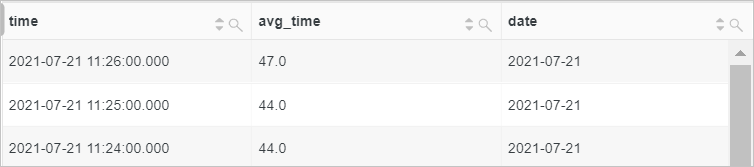The date_add function adds a specified interval to or subtract a specified interval from a date or time.

### Syntax

``date_add(unit, n,x)``

### Parameters

Parameter Description
unit The unit of time. Valid values: millisecond, second, minute, hour, day, week, month, quarter, and year. For more information, see Units.
n The time interval.
x The value of this parameter is a datetime expression that can return a timestamp value.

### Return value type

The timestamp type.

### Examples

Query the logs of the previous day.

• Query statement
``````* |
SELECT
*
FROM  log
WHERE
__time__ < to_unixtime(current_timestamp)
AND __time__ > to_unixtime(date_add('day', -1, current_timestamp))``````
• Query and analysis results## date_diff function

The date_diff function returns the difference between two dates or points in time.

### Syntax

``date_diff(unit, x, y)``

### Parameters

Parameter Description
unit The unit of time. Valid values: millisecond, second, minute, hour, day, week, month, quarter, and year. For more information, see Units.
x The value of this parameter is a datetime expression that can return a timestamp value.
y The value of this parameter is a datetime expression that can return a timestamp value.

The bigint type.

### Examples

Calculate the runtime duration of a server based on the UsageStartTime and UsageEndTime fields.

• Query statement
``* | SELECT  date_diff('hour', UsageStartTime, UsageEndTime) AS "Time difference (hour)"``
• Query and analysis results## time_series function

The time_series function adds a value to the field that has no value returned in the specified time window.
Notice You must use the time_series function together with the GROUP BY and ORDER BY clauses. You cannot use the DESC keyword in the ORDER BY clause to sort data.

### Syntax

``time_series(x, window_time, format, padding_data)``

### Parameters

Parameter Description
x The time column. Example: __time__. The value of this parameter is of the long or timestamp type.
window_time The duration of the time window. Unit: s, m, h, and d. s indicates second, m indicates minute, h indicates hour, and d indicates day. Examples: 2h, 5m, and 3d.
format The time format in which you want the function to return the value. For more information, see Formats.
• 0: The value 0 is added.
• null: The value null is added.
• last: The value of the previous point in time is added.
• next: The value of the next point in time is added.
• avg: The average value of the previous point in time and the next point in time is added.

### Return value type

The varchar type.

### Examples

Add the value 0 to the fields that have no value returned during two hours.

• Query statement
``* | select time_series(__time__, '2h', '%Y-%m-%d %H:%i:%s', '0')  as time, count(*) as num from log group by time order by time                        ``
• Query and analysis results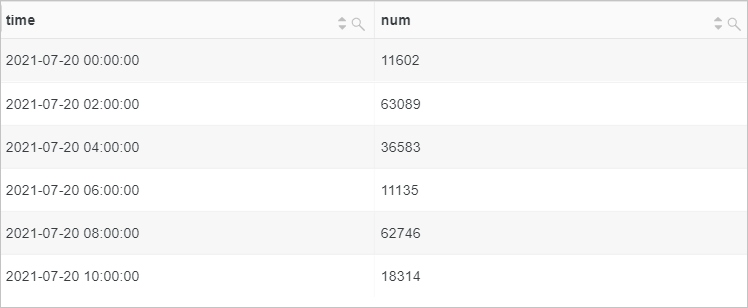## References

• Formats
Format Description
%a The abbreviation for the day of the week. Examples: Sun and Sat.
%b The abbreviation for the month of the year. Examples: Jan and Dec.
%c The month. The value is of the numeric type. Valid values: 1 to 12.
%D The day of the month. Examples: 0th, 1st, 2nd, and 3rd.
%d The day of the month. The value is in the decimal format. Valid values: 01 to 31.
%e The day of the month. The value is in the decimal format. Valid values: 1 to 31.
%H The hour. The 24-hour clock is used.
%h The hour. The 12-hour clock is used.
%I The hour. The 12-hour clock is used.
%i The minute. The value is of the numeric type. Valid values: 00 to 59.
%j The day of the year. Valid values: 001 to 366.
%k The hour. Valid values: 0 to 23.
%l The hour. Valid values: 1 to 12.
%M The full month name. Examples: January and December.
%m The month. The value is of the numeric type. Valid values: 01 to 12.
%p The abbreviation that indicates the morning or afternoon of the day. Valid values: AM and PM.
%r The time. The 12-hour clock is used. The time is in the `hh:mm:ss AM/PM` format.
%S The second. Valid values: 00 to 59.
%s The second. Valid values: 00 to 59.
%T The time. The 24-hour clock is used. The time is in the `hh:mm:ss` format.
%V The week of the year. Sunday is the first day of a week. Valid values: 01 to 53.
%v The week of the year. Monday is the first day of a week. Valid values: 01 to 53.
%W The full name of the day of the week. Examples: Sunday and Saturday.
%w The day of the week. The value 0 indicates Sunday.
%Y The four-digit year. Example: 2020.
%y The two-digit year. Example: 20.
%% The escape character of the percent sign (%).
• Units
Unit Description
millisecond Millisecond
second Second
minute Minute
hour Hour
day Day
week Week
month Month
quarter Quarter
year Year